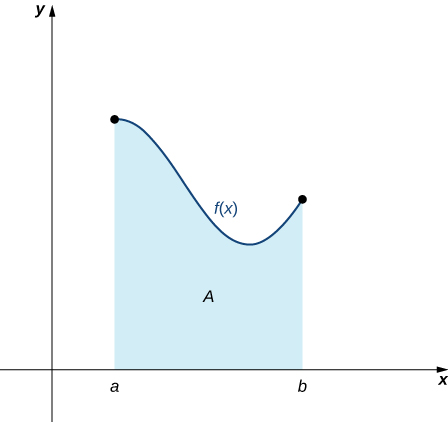# 5.1 Approximating areas  (Page 2/17)

 Page 2 / 17

Write in sigma notation and evaluate the sum of terms 2 i for $i=3,4,5,6.$

$\sum _{i=3}^{6}{2}^{i}={2}^{3}+{2}^{4}+{2}^{5}+{2}^{6}=120$

The properties associated with the summation process are given in the following rule.

## Rule: properties of sigma notation

Let ${a}_{1},{a}_{2}\text{,…,}\phantom{\rule{0.2em}{0ex}}{a}_{n}$ and ${b}_{1},{b}_{2}\text{,…,}\phantom{\rule{0.2em}{0ex}}{b}_{n}$ represent two sequences of terms and let c be a constant. The following properties hold for all positive integers n and for integers m , with $1\le m\le n.$

1. $\sum _{i=1}^{n}c=nc$

2. $\sum _{i=1}^{n}c{a}_{i}=c\sum _{i=1}^{n}{a}_{i}$

3. $\sum _{i=1}^{n}\left({a}_{i}+{b}_{i}\right)=\sum _{i=1}^{n}{a}_{i}+\sum _{i=1}^{n}{b}_{i}$

4. $\sum _{i=1}^{n}\left({a}_{i}-{b}_{i}\right)=\sum _{i=1}^{n}{a}_{i}-\sum _{i=1}^{n}{b}_{i}$

5. $\sum _{i=1}^{n}{a}_{i}=\sum _{i=1}^{m}{a}_{i}+\sum _{i=m+1}^{n}{a}_{i}$

## Proof

We prove properties 2. and 3. here, and leave proof of the other properties to the Exercises.

2. We have

$\begin{array}{cc}\sum _{i=1}^{n}c{a}_{i}\hfill & =c{a}_{1}+c{a}_{2}+c{a}_{3}+\text{⋯}+c{a}_{n}\hfill \\ & =c\left({a}_{1}+{a}_{2}+{a}_{3}+\text{⋯}+{a}_{n}\right)\hfill \\ \\ \\ & =c\sum _{i=1}^{n}{a}_{i}.\hfill \end{array}$

3. We have

$\begin{array}{cc}\sum _{i=1}^{n}\left({a}_{i}+{b}_{i}\right)\hfill & =\left({a}_{1}+{b}_{1}\right)+\left({a}_{2}+{b}_{2}\right)+\left({a}_{3}+{b}_{3}\right)+\text{⋯}+\left({a}_{n}+{b}_{n}\right)\hfill \\ & =\left({a}_{1}+{a}_{2}+{a}_{3}+\text{⋯}+{a}_{n}\right)+\left({b}_{1}+{b}_{2}+{b}_{3}+\text{⋯}+{b}_{n}\right)\hfill \\ \\ \\ & =\sum _{i=1}^{n}{a}_{i}+\sum _{i=1}^{n}{b}_{i}.\hfill \end{array}$

A few more formulas for frequently found functions simplify the summation process further. These are shown in the next rule, for sums and powers of integers , and we use them in the next set of examples.

## Rule: sums and powers of integers

1. The sum of n integers is given by
$\sum _{i=1}^{n}i=1+2+\text{⋯}+n=\frac{n\left(n+1\right)}{2}.$
2. The sum of consecutive integers squared is given by
$\sum _{i=1}^{n}{i}^{2}={1}^{2}+{2}^{2}+\text{⋯}+{n}^{2}=\frac{n\left(n+1\right)\left(2n+1\right)}{6}.$
3. The sum of consecutive integers cubed is given by
$\sum _{i=1}^{n}{i}^{3}={1}^{3}+{2}^{3}+\text{⋯}+{n}^{3}=\frac{{n}^{2}{\left(n+1\right)}^{2}}{4}.$

## Evaluation using sigma notation

Write using sigma notation and evaluate:

1. The sum of the terms ${\left(i-3\right)}^{2}$ for $i=1,2\text{,…,}\phantom{\rule{0.2em}{0ex}}200.$
2. The sum of the terms $\left({i}^{3}-{i}^{2}\right)$ for $i=1,2,3,4,5,6.$
1. Multiplying out ${\left(i-3\right)}^{2},$ we can break the expression into three terms.
$\begin{array}{cc}\sum _{i=1}^{200}{\left(i-3\right)}^{2}\hfill & =\sum _{i=1}^{200}\left({i}^{2}-6i+9\right)\hfill \\ \\ \\ & =\sum _{i=1}^{200}{i}^{2}-\sum _{i=1}^{200}6i+\sum _{i=1}^{200}9\hfill \\ & =\sum _{i=1}^{200}{i}^{2}-6\sum _{i=1}^{200}i+\sum _{i=1}^{200}9\hfill \\ & =\frac{200\left(200+1\right)\left(400+1\right)}{6}-6\left[\frac{200\left(200+1\right)}{2}\right]+9\left(200\right)\hfill \\ & =2,686,700-120,600+1800\hfill \\ & =2,567,900\hfill \end{array}$
2. Use sigma notation property iv. and the rules for the sum of squared terms and the sum of cubed terms.
$\begin{array}{cc}\sum _{i=1}^{6}\left({i}^{3}-{i}^{2}\right)\hfill & =\sum _{i=1}^{6}{i}^{3}-\sum _{i=1}^{6}{i}^{2}\hfill \\ \\ \\ \\ & =\frac{{6}^{2}{\left(6+1\right)}^{2}}{4}-\frac{6\left(6+1\right)\left(2\left(6\right)+1\right)}{6}\hfill \\ & =\frac{1764}{4}-\frac{546}{6}\hfill \\ & =350\hfill \end{array}$

Find the sum of the values of $4+3i$ for $i=1,2\text{,…,}\phantom{\rule{0.2em}{0ex}}100.$

15,550

## Finding the sum of the function values

Find the sum of the values of $f\left(x\right)={x}^{3}$ over the integers $1,2,3\text{,…,}\phantom{\rule{0.2em}{0ex}}10.$

Using the formula, we have

$\begin{array}{cc}\sum _{i=0}^{10}{i}^{3}\hfill & =\frac{{\left(10\right)}^{2}{\left(10+1\right)}^{2}}{4}\hfill \\ \\ & =\frac{100\left(121\right)}{4}\hfill \\ & =3025.\hfill \end{array}$

Evaluate the sum indicated by the notation $\sum _{k=1}^{20}\left(2k+1\right).$

440

## Approximating area

Now that we have the necessary notation, we return to the problem at hand: approximating the area under a curve. Let $f\left(x\right)$ be a continuous, nonnegative function defined on the closed interval $\left[a,b\right].$ We want to approximate the area A bounded by $f\left(x\right)$ above, the x -axis below, the line $x=a$ on the left, and the line $x=b$ on the right ( [link] ).An area (shaded region) bounded by the curve f ( x ) at top, the x -axis at bottom, the line x = a to the left, and the line x = b at right.

How do we approximate the area under this curve? The approach is a geometric one. By dividing a region into many small shapes that have known area formulas, we can sum these areas and obtain a reasonable estimate of the true area. We begin by dividing the interval $\left[a,b\right]$ into n subintervals of equal width, $\frac{b-a}{n}.$ We do this by selecting equally spaced points ${x}_{0},{x}_{1},{x}_{2}\text{,…,}\phantom{\rule{0.2em}{0ex}}{x}_{n}$ with ${x}_{0}=a,{x}_{n}=b,$ and

${x}_{i}-{x}_{i-1}=\frac{b-a}{n}$

for $i=1,2,3\text{,…,}\phantom{\rule{0.2em}{0ex}}n.$

We denote the width of each subinterval with the notation Δ x , so $\text{Δ}x=\frac{b-a}{n}$ and

${x}_{i}={x}_{0}+i\text{Δ}x$

#### Questions & Answers

Find the derivative of g(x)=−3.
Abdullah Reply
any genius online ? I need help!!
Guzorochi Reply
how can i help you?
Pina
need to learn polynomial
Zakariya
i will teach...
nandu
I'm waiting
Zakariya
plz help me in question
Abish
evaluate the following computation (x³-8/x-2)
Murtala Reply
teach me how to solve the first law of calculus.
Uncle Reply
what is differentiation
Ibrahim Reply
f(x) = x-2 g(x) = 3x + 5 fog(x)? f(x)/g(x)
Naufal Reply
fog(x)= f(g(x)) = x-2 = 3x+5-2 = 3x+3 f(x)/g(x)= x-2/3x+5
diron
pweding paturo nsa calculus?
jimmy
how to use fundamental theorem to solve exponential
JULIA Reply
find the bounded area of the parabola y^2=4x and y=16x
Omar Reply
what is absolute value means?
Geo Reply
Chicken nuggets
Hugh
🐔
MM
🐔🦃 nuggets
MM
(mathematics) For a complex number a+bi, the principal square root of the sum of the squares of its real and imaginary parts, √a2+b2 . Denoted by | |. The absolute value |x| of a real number x is √x2 , which is equal to x if x is non-negative, and −x if x is negative.
Ismael
find integration of loge x
Game Reply
find the volume of a solid about the y-axis, x=0, x=1, y=0, y=7+x^3
Godwin Reply
how does this work
Brad Reply
Can calculus give the answers as same as other methods give in basic classes while solving the numericals?
Cosmos Reply
log tan (x/4+x/2)
Rohan
please answer
Rohan
y=(x^2 + 3x).(eipix)
Claudia
is this a answer
Ismael
A Function F(X)=Sinx+cosx is odd or even?
WIZARD Reply
neither
David
Neither
Lovuyiso
f(x)=1/1+x^2 |=[-3,1]
Yuliana Reply
apa itu?
fauzi

### Read also:

#### Get Jobilize Job Search Mobile App in your pocket Now!

Source:  OpenStax, Calculus volume 1. OpenStax CNX. Feb 05, 2016 Download for free at http://cnx.org/content/col11964/1.2
Google Play and the Google Play logo are trademarks of Google Inc.

Notification Switch

Would you like to follow the 'Calculus volume 1' conversation and receive update notifications?ByBy Dionne MahaffeyBy Brooke DelaneyBy Rachel CarlisleBy Brooke DelaneyBy OpenStaxBy Richley CrapoBy Anonymous UserBy Mariah HauptmanBy Ali Sid